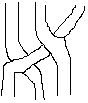Recall that $2 \times 2$ spin matrices are associated with the quantum Fourier transform for$q = -1$. The Weyl rule $UV = - VU$ may be thought of as a square with paired edges marked $U$ and $V$, just like in the planar paths considered by Kapranov. In 3 dimensions one draws paths on a cubic lattice. The paths on a single cube form the vertices of one of our favourite hexagons. A simple braid on three strands is formed by composing two edges of this hexagon, which correspond to two faces on the cube. Since the Weyl edges $U$ and $V$ have become faces in 3D, this composition can represent fermionic spin, just as Bilson-Thompson said.